# Creating Your First Calculated Field

To create a calculated field, first activate the Insert Calculated Field dialog box shown in Figure 6.6. To call up this dialog box, place your cursor anywhere in your pivot table, click the PivotTable icon in the PivotTable toolbar, and then select Formulas, Calculated Field.

##### Figure 6.6. The Insert Calculated Field dialog box will assist you in creating a calculated field in your pivot table.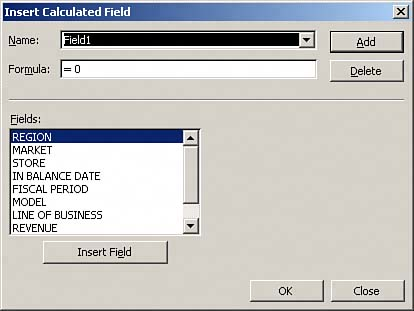NOTE

Within Office 2000, right-click on your pivot table and select Formulas, Calculated Field to get to the Insert Calculation Field dialog box.

TIP

If your PivotTable toolbar is active, you can click anywhere inside your pivot table and press Alt+P to see all the menu items under the PivotTable icon selection.

You will notice the two input boxes at the top of the dialog box shown in Figure 6.7: Name and Formula. The objective here is to give your calculated field a name and then build the formula by selecting the combination of data fields and mathematical operators that will provide you the metric you are looking for. In this example, you will first name your calculated field "Avg Revenue per Unit."

##### Figure 6.7. Give your calculated field a descriptive name.Next, you will go to the Fields list, as shown in Figure 6.8, and double-click on the Revenue field. Then enter a slash (/) to let Excel know you will be dividing the Revenue field by something.

##### Figure 6.8. Start your formula with = Revenue /.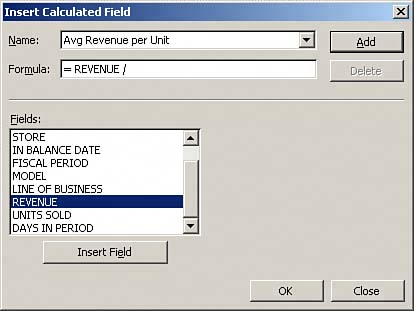CAUTION

By default, the Formula input box in the Insert Calculated Field dialog box contains "= 0". Ensure that you delete the zero before continuing with your formula.

Finish your formula by double-clicking on the Units Sold field. Your finished formula is shown in Figure 6.9.

##### Figure 6.9. The full formula, = Revenue / 'Units Sold', will give you the calculated field you need.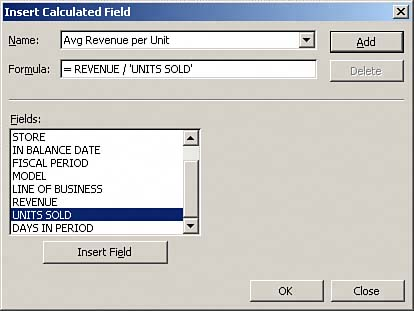Click the Add button and then click OK to activate your new calculated field. As you can see in Figure 6.10, the result of your efforts is a calculated field within your pivot table.

##### Figure 6.10. You have successfully added a calculated field to your pivot table. You may now change the settings on this new field just as you would any other field (for example, you could change the field name, the number format, or the color).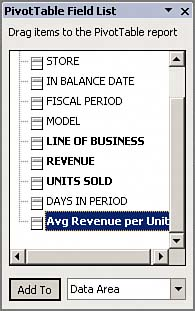Notice that not only did your pivot table create a new field called Sum of Avg Revenue per Unit, but your field list now includes your new calculated field.

Does this mean you have just added a column to your data source? The answer is no. Calculated fields are similar to the pivot table's default Subtotal and Grand Total calculations in that they are all mathematical functions that recalculate when the pivot table changes or is refreshed. Calculated fields merely mimic the hard fields in your data source, allowing you to drag them, change field settings, and use them with other calculated fields.

Take a moment to look at Figure 6.9 closely. Notice the formula you entered is similar in format to those used in the standard Excel formula bar. The obvious difference is that instead of using hard numbers or cell references, you are referencing pivot data fields to define the arguments used in this calculation. If you have worked with formulas in Excel before, you will quickly grasp the concept of creating calculated fields.

#### CASE STUDY: Summarizing Next Year's Forecast

Each store in your company has submitted its initial revenue forecast for next year. Your task is to take the first-pass numbers the stores submitted and create a summary report showing the following:

• Total revenue forecast by region and market

• Total percentage growth over last year

• Total contribution margin by region and market

Because these numbers are first-pass submissions and you know they will change over the course of the next two weeks, you decide to use a pivot table to create the requested forecast summary from the source data shown in Figure 6.11.

##### Figure 6.11. Using a pivot table will give you the flexibility to update your summary report without re-creating it.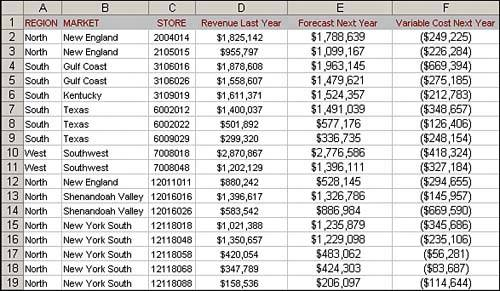Create the initial pivot table to include the fields Revenue Last Year and Forecast Next Year for each region and market.

As you can see in Figure 6.12, by virtue of adding the Forecast Next Year field in the data area, you have met your first requirementto show the total revenue forecast by region and market.

##### Figure 6.12. The initial pivot table is basic, but it provides the data for your first requirementto show the total revenue forecast by region and market.The next metric you need is percentage growth over last year. To get this data, you will need to add a calculated field that uses the following formula:

(Forecast Next Year - Revenue Last Year) / 'Revenue Last Year'

Click the PivotTable icon in the PivotTable toolbar and then select Formulas, Calculated Field. When you have activated the Insert Calculated Field dialog box, name your new field Percent Growth.

Activate the Insert Calculated Field dialog box and name your new field Percent Growth.

Delete the zero that appears in the Formula input box and then follow these steps:

 1 Enter an opening parenthesis [(]. 2 Double-click on the Forecast Next Year field. 3 Enter a minus sign (-). 4 Double-click on the Revenue Last Year field. 5 Enter a closing parenthesis [)]. 6 Enter a division sign (/). 7 Double-click on the Revenue Last Year field.

At this point, your dialog box should look similar to the one shown in Figure 6.13. With your formula typed in, you can now click OK to add your new field.

##### Figure 6.13. With just a few clicks, you have created your percentage growth formula.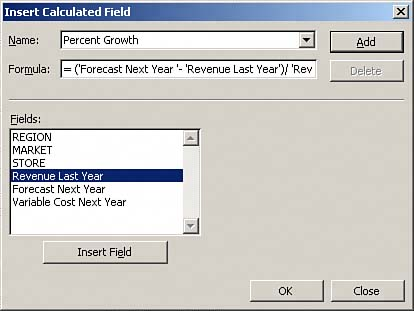As you can see in Figure 6.14, the default format of your calculated field makes it look like there is zero growth across the board. You will often find that it is necessary to format your newly calculated fields to present your data properly. In this case, you will have to go into your new field's settings and format it to show your data as percentages.

##### Figure 6.14. Some formatting is needed to show data correctly.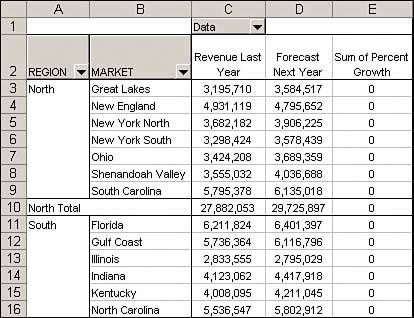After you have formatted your calculated field, your pivot table should look similar to the one shown in Figure 6.15. With your new calculated field in place, you can easily see that the New England market and the Illinois market will have to resubmit their forecasts to reflect a positive growth over last year.

##### Figure 6.15. You can already discern some information from your calculated field, identifying New England and Illinois as problem markets.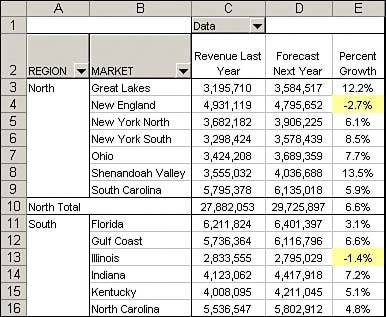Now that you show total revenue forecast by region and market as well as the total percentage growth over last year, it's time to focus on your last requirementto show the total contribution margin by region and market.

To get this data, you will need to add a calculated field that uses the following formula:

Forecast Next Year + Variable Cost Next Year

NOTE

By looking at Figure 6.15, you can see that Variable Cost Next Year is not in your pivot table report. Your calculated field can use fields that are not visible in your pivot table.

Activate the Insert Calculated Field dialog box and then name your new field Contribution Margin.

Delete the zero that appears in the Formula input box and then follow these steps:

 1 Double-click on the Forecast Next Year field. 2 Enter a plus sign (+). 3 Double-click on the Variable Cost Next Year field.

At this point, your dialog box should look similar to the one shown in Figure 6.16.

##### Figure 6.16. With just a few clicks, you have created your contribution margin formula.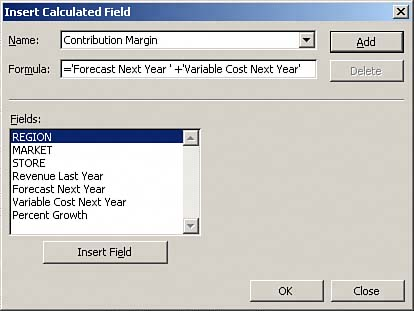With the creation of the contribution margin, your pivot table report should look similar to the one shown in Figure 6.17. This report is ready to be delivered.

##### Figure 6.17. Contribution margin is now a data field in your pivot table report thanks to your calculated field.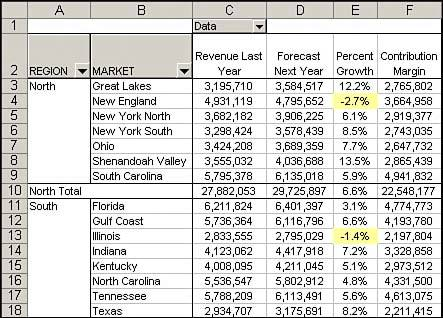Now that your pivot table report is built, any new forecast submissions can easily be analyzed by refreshing your report with the new updates.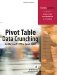Pivot Table Data Crunching for Microsoft Office Excel 2007
ISBN: 0789736012
EAN: 2147483647
Year: 2003
Pages: 140

Similar book on Amazon